Question

A vehicle of mass 2000 kg has a constant resistance to motion of 3000 N. The maximum speed of the vehicle, when travelling on a horizontal road, is 120 km/hour. Find the acceleration of the car when it is travelling at a speed of 50 km/hour up a hill inclined at an angle of 3o to the horizontal, with the engine operating at maximum power (as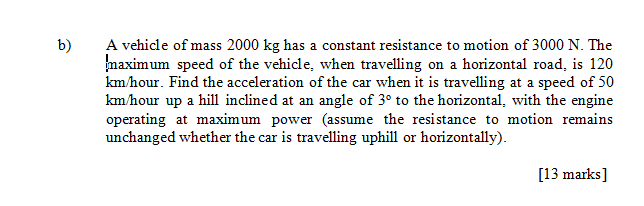sume the resistance to motion remains unchanged whether the car is travelling uphill or horizonally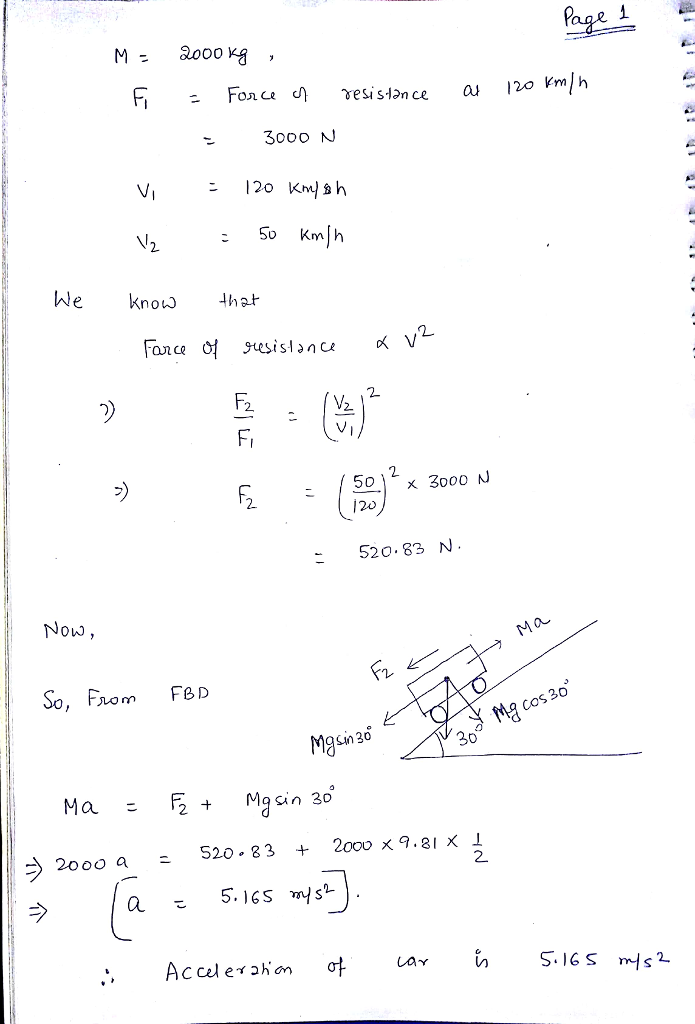#### Earn Coins

Coins can be redeemed for fabulous gifts.

Similar Homework Help Questions
• ### 1 The motorcycle has a mass of 750 kg and can maintain a steady speed of...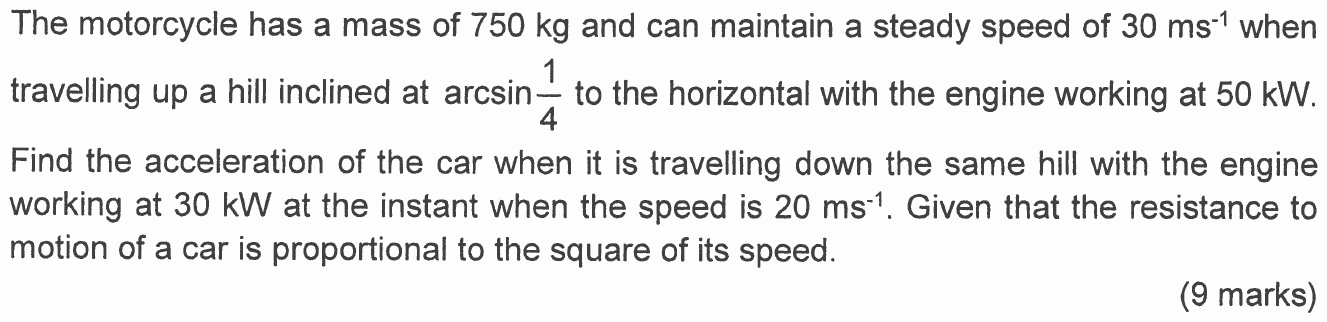1 The motorcycle has a mass of 750 kg and can maintain a steady speed of 30 ms-t when travelling up a hill inclined at arcsin to the horizontal with the engine working at 50 kW. 4 Find the acceleration of the car when it is travelling down the same hill with the engine working at 30 kW at the instant when the speed is 20 ms-1. Given that the resistance to motion of a car is proportional to the...

• ### 21) The following figures apply to a certain car running in top gear on a level...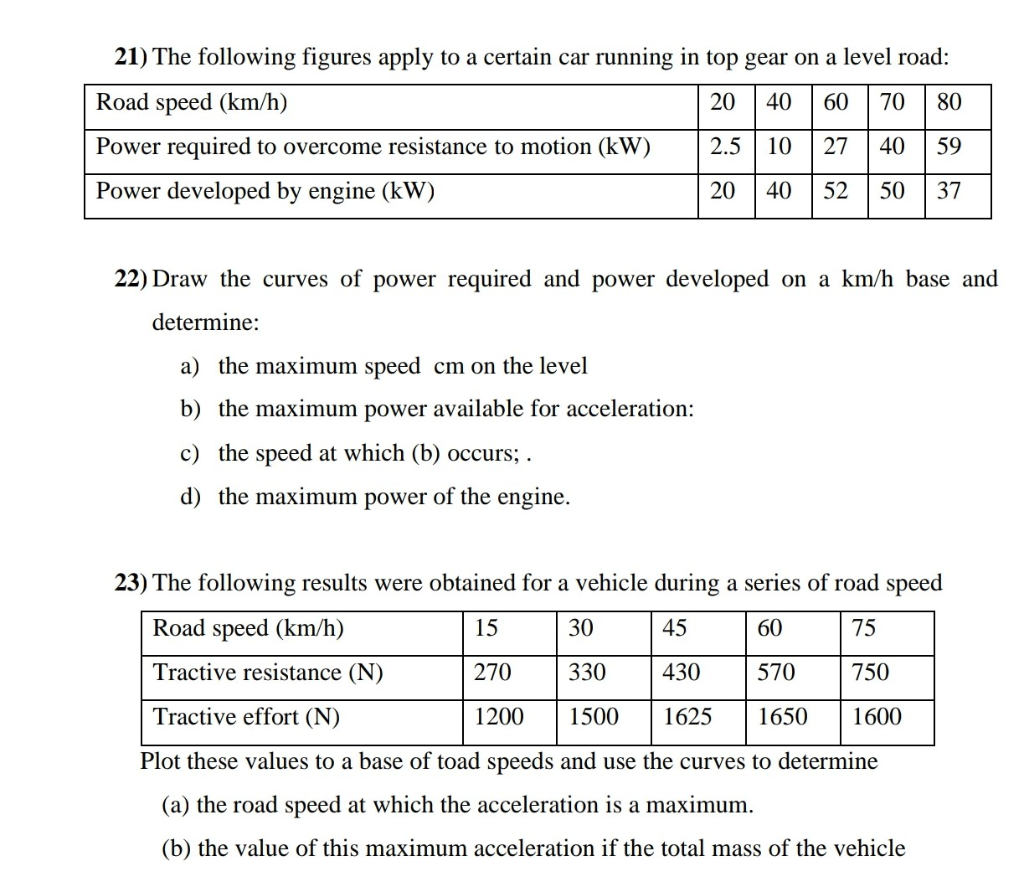21) The following figures apply to a certain car running in top gear on a level road: Road speed (km/h) 20 40 60 70 80 Power required to overcome resistance to motion (kW) 2.5 10 27 40 59 Power developed by engine (kW) 20 40 52 50 37 22) Draw the curves of power required and power developed on a km/h base and determine: a) the maximum speed cm on the level b) the maximum power available for acceleration: c)...

• ### Finding Power and Resistance?

A cyclist and her bicycle have a total mass of #84kg#. She works at a constant rate of #P# #W# while moving on a straight road which is inclined to the horizontal at an angle #theta#, where #sintheta = 0.1#. When moving uphill, the cyclist's acceleration is #1.25m#/#s^2# at an instant when her speed is #3m#/#s^1#. When moving downhill, the cyclist's acceleration is #1.25m#/#s^2# at an instant when her speed is #10m#/#s^1#. The resistance to the cyclist's motion, whether the...

• ### a 1800 kg car experiences a combined force of air resistance and friction that has the...

a 1800 kg car experiences a combined force of air resistance and friction that has the same magnitude whether the car goes up or down a hill at 24 m/s. going up a hill, the cars engine produces 44 hp more power to sustain the constant velocity than it does going down the same hill. At what angle is the hill inclined above the horizontal?

• ### 2. The electric vehicle Tesla Model S has a drag coefficient of 0.24, a mass of 2100 kg, and a frontal area of 2.4 m2....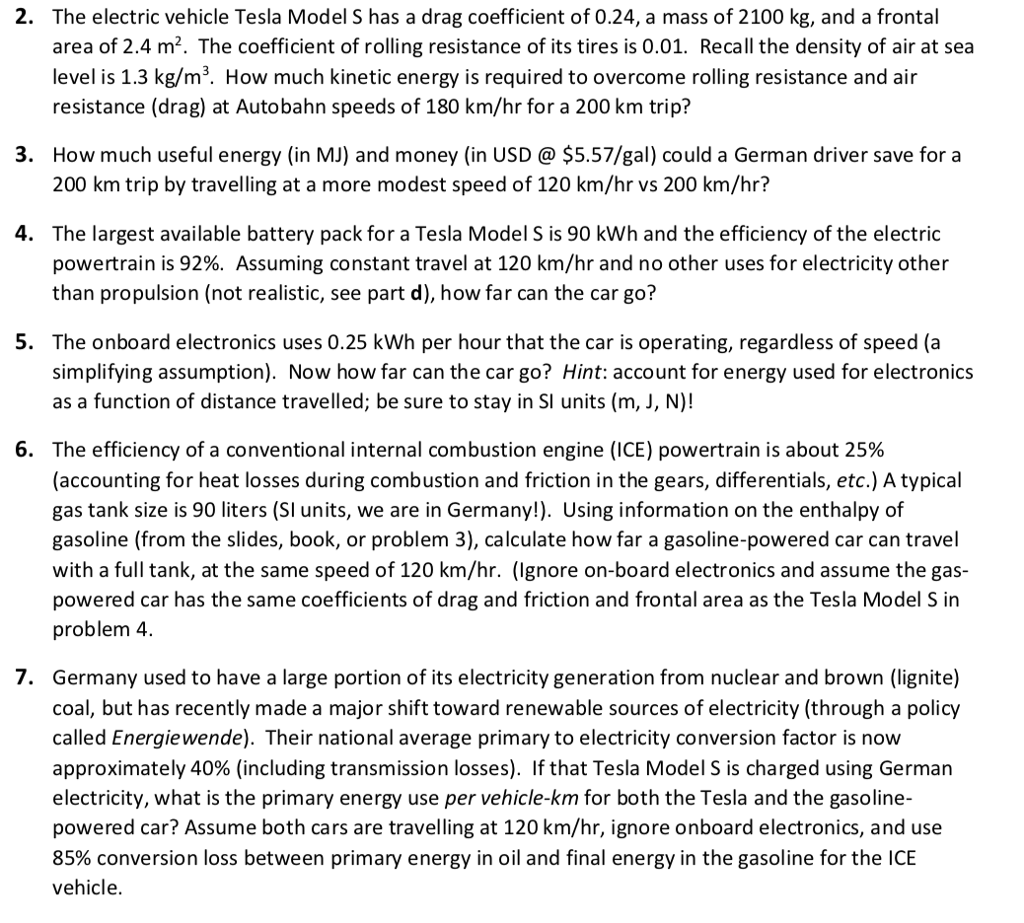2. The electric vehicle Tesla Model S has a drag coefficient of 0.24, a mass of 2100 kg, and a frontal area of 2.4 m2. The coefficient of rolling resistance of its tires is 0.01. Recall the density of air at sea level is 1.3 kg/m3. How much kinetic energy is required to overcome rolling resistance and air resistance (drag) at Autobahn speeds of 180 km/hr for a 200 km trip? How much useful energy (in MJ) and money (in...

• ### A car of mass 1200kg pulls a trailer of mass of 400kg along a straight horizontal road. The car and trailer are connected by a tow-rope modelled as a light inextensible rod. The engine of the car provides a constant driving force of 3200N. (see ? below)

The horizontal resistances of the car and the trailer are proportional to their respective masses. Given that the acceleration of the car and trailer is 0.4ms-2, a) find the resistance to motion on the trailer b) find the tension in the tow-rope When the car and trailer are travelling at 25ms-1 the tow-rope breaks. Assuming the resistances to motion are unchanged, c) find the distance the trailer travels before coming to a stop

• ### Problem09 Cicular Motion of a car moving with uniform.speed A car with mass (m-300 Kg) is...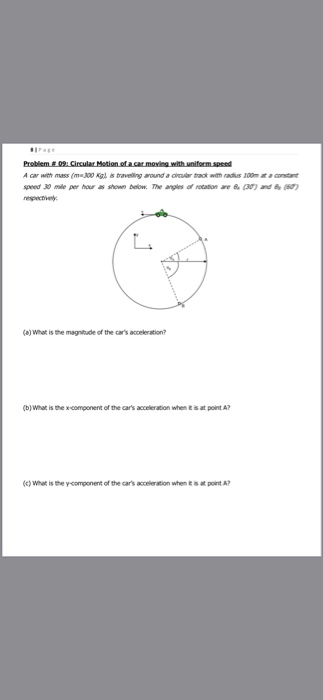Problem09 Cicular Motion of a car moving with uniform.speed A car with mass (m-300 Kg) is travelling around a croular track with radus 100m ar a constant speed 30 mile per hour as shown below. The angles of rotation are (30) and 6 (60) respectively (a)What is the magnitude of the car's acceleration? (b)What is the x-component of the car's acceleration when it is at point A? (What is the y-component of the car's acceleration when it is at point...

• ### Problem 1 (50): A 3100 lb car has a 5-speed manual transmission with i;= 3.22, 12...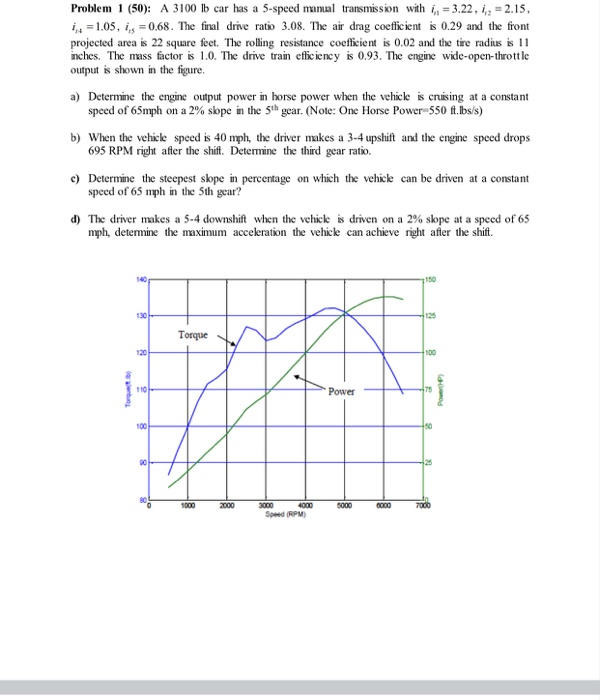Problem 1 (50): A 3100 lb car has a 5-speed manual transmission with i;= 3.22, 12 = 2.15, 14 = 1.05, 1,5 = 0.68. The final drive ratio 3.08. The air drag coefficient is 0.29 and the front projected area is 22 square feet. The rolling resistance coefficient is 0.02 and the tire radius is 11 inches. The mass factor is 1.0. The drive train efficiency is 0.93. The engine wide-open-throttle output is shown in the figure, a) Determine the...

• ### Problem 1 (50): A 3100 lb car has a 5-speed manual transmission with in = 3.22,...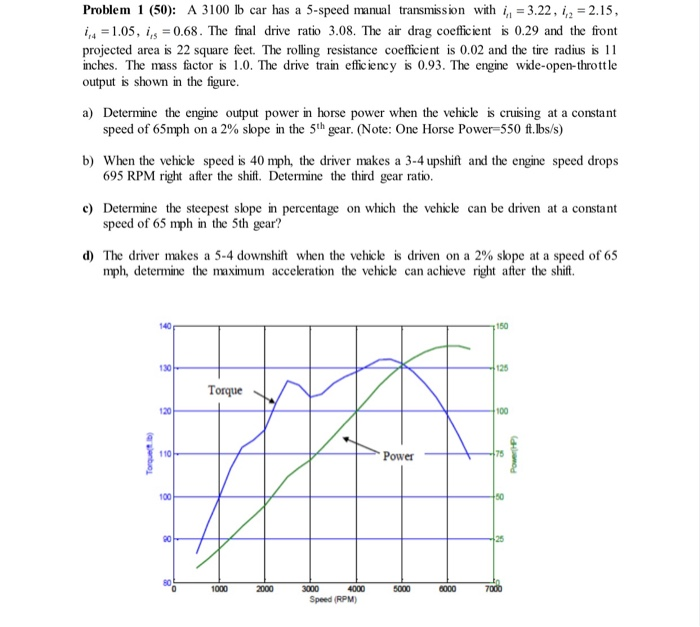Problem 1 (50): A 3100 lb car has a 5-speed manual transmission with in = 3.22, 1,2 = 2.15, 1,4 = 1.05, 1,5 = 0.68. The final drive ratio 3.08. The air drag coefficient is 0.29 and the front projected area is 22 square feet. The rolling resistance coefficient is 0.02 and the tire radius is 11 inches. The mass factor is 1.0. The drive train efficiency is 0.93. The engine wide-open-throttle output is shown in the figure. a) Determine...

• ### Please help asap with this. I will rate with a thumbs up. Problem 1 (50): A...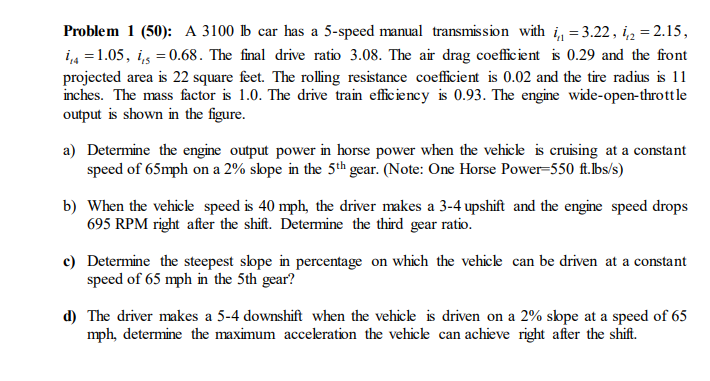Please help asap with this. I will rate with a thumbs up. Problem 1 (50): A 3100 b car has a 5-speed manual transmission with in = 3.22, 1,2 = 2.15, 1,4 = 1.05, 1,5 = 0.68. The final drive ratio 3.08. The ar drag coefficient is 0.29 and the front projected area is 22 square feet. The rolling resistance coefficient is 0.02 and the tire radius is 11 inches. The mass factor is 1.0. The drive train efficiency is...